CAT Quant practice questions, trigonometry practice - Ascent Education - CAT correspondence programs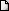LATEST NEWS • Ascent Success Stories • TANCET 16 Classes @ Chennai • XAT 16 Classes @ Chennai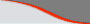Home | TANCET Coaching Class | TANCET study material | Online TANCET Course | Contact us
 Ascent's Courses CAT 2010 for IIMs CAT Classroom Chennai Math & DI Crash Course CAT - Correspondence CAT Correspondence India CAT Corres Abroad Mock CAT Series CAT Crash Course Math Shortcut Workshops XAT Classes TANCET 2010 Classes MAT Classes GRE-GMAT GMAT Classes US B Schools Faculty List Question A Day Business Partnership Jobs @ Ascent Testimonials Ascent TANCET Toppers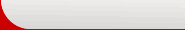### Trigonometric ratios - Quant/Math - CAT 2016

Question 4 the day: July 02, 2002

The question for the day is a quant problem from the topic of Trigonometric ratios.

Question:
The angle of elevation of the top of a tower 30 m high, from two points on the level ground on its opposite sides are 45 degrees and 60 degrees. What is the distance between the two points?
(1) 30
(2) 51.96
(3) 47.32
(4) 81.96
Correct choice (3). Correct Answer - 47.32

Solution: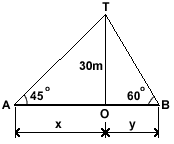Let OT be te tower.

Therefore, Height of tower = OT = 30 m
Let A and B be the two points on the level ground on the opposite side of tower OT.

Then,
angle of elevation from A =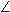TAO = 45o
and angle of elevation from B =TBO = 60o
Distance between AB = AO + OB = x + y (say)
Now, in right triangle ATO,
tan 45o =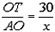=> x == 30 m
and in right traingle BTO
tan 60o ==> y =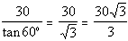= 17.32 m

Hence, the required distance = x + y = 30 + 17.32 = 47.32 m

## XLRI XAT TANCET Practice Question Samples

#### Question Archives

GMAT Math Books
 Comprehensive study material for GMAT Quant. 1800+ questions.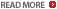CAT Online Course

GMAT Math eBook Series
 GMAT Math eBooks series on high scoring topics such as permutation combination, number theory, geometry. US\$ 6.99.GMAT Math Lesson Book | SAT prep | GRE Classes @ Chennai | GMAT Coaching @ Chennai | TANCET Mailing List © 2002 - 17 ASCENT Education all rights reserved. Terms & Condition | Privacy Policy